## ZEROCROSSING

 Applies a zero crossing edge detector to an image.

 USAGE: zerocrossing [-w width ] [-b brightness] [-s smoothing] [-g] infile outfile USAGE: zerocrossing [-h or -help] -e ... edge ...... edge type; choices are sobel (s) or morphologic (m); .................. default=sobel -l ... laplace ... laplacian type; choices are: 0, 2 and 5; default=0 -a ... amplify ... zero crossing percent amplification; integer>=0; .................. default=100 (no amplification) -s ... size ...... 2x morphologic disk size; values are integer>=2; .................. default=4 (values will be divided by 2 later) -g ............... convert image to grayscale before getting edges PURPOSE: To apply a zero crossing edge detector to image. DESCRIPTION: ZEROCROSSING applies a zero crossing edge detector to image in order to thin the edges. The user may select either sobel or morphologic for the basic type of edge detection as well as the type of laplacian used for detecting the zero crossings. ARGUMENTS: -e edge ... EDGE type. The choices are sobel (s) or morphologic (m). The default=sobel. -l laplace ... LAPLACIAN type. The choices are: 0, 2 and 5. The default=0. -a amplify ... AMPLIFICATION is the zero crossing percent amplification. Values are integer>=0. The default=100 (no amplification). -s size ... SIZE is twice the morphologic disk size. Values are integer>=2. The default=4 (values will be divided by 2 later). -g ... converts the input image to GRAYSCALE before extracting edges. REQUIREMENTS: IM 6.5.9.3 is required for the use of -morphology edges. IM 6.6.1.8 is required for the use of sobel edges. CAVEAT: No guarantee that this script will work on all platforms, nor that trapping of inconsistent parameters is complete and foolproof. Use At Your Own Risk.

### EXAMPLES

 Example 1 -- Sobel Gray Original SobelArguments: -e sobel -l 0 -g Arguments: -e sobel -l 2 -g Arguments: -e sobel -l 5 -g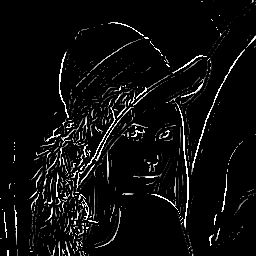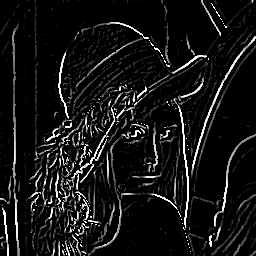Arguments: -e sobel -l 5 -a 400 -gExample 2 -- Morphologic Gray Original Mophologic (size=4)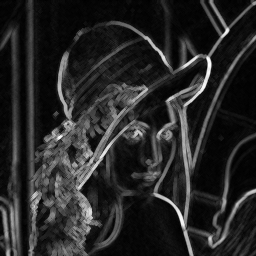Arguments: -e morphologic -s 4 -l 0 -g Arguments: -e morphologic -s 4 -l 2 -g Arguments: -e morphologic -s 4 -l 4 -gArguments: -e sobel -l 5 -s 8 -a 100 -g Arguments: -e sobel -l 5 -a 400 -g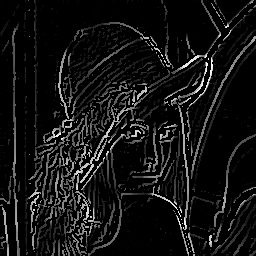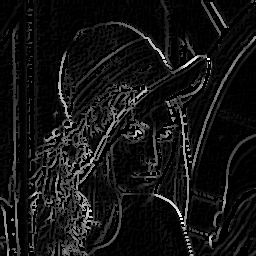Example 3 -- Color Original Sobel Mophologic (size=4)Arguments: -e sobel -l 5 -a 400 Arguments: -e morphologic -l 5 -a 400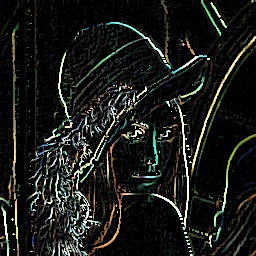What the script does is as follows: Applies either a sobel or mophologic edge detector to the input image Applies a laplacian edge detector to the input image Applies a gradient magnitude to the laplacian image and thresholds to find the zero crossings. Multiplies the zero crossing (mask) image with the edge detected image. This is equivalent to the following IM commands convert -quiet -regard-warnings "\$infile" +repage "\$tmpA1" if [ "\$grayscale" = "yes" ]; then gproc="-colorspace gray" else gproc="" fi size=`convert xc: -format "%[fx:\$size/2]" info:` if [ "\$edge" = "sobel" ]; then eproc="-define convolve:scale='!' -define morphology:compose=Lighten -morphology Convolve Sobel:>" elif [ "\$edge" = "morphologic" ]; then eproc="-morphology edge disk:\$size -auto-level " fi convert \$tmpA1 \$gproc \ \( -clone 0 \$eproc \) \ \( -clone 0 -bias 50% -morphology Convolve Laplacian:\$laplace \) \ \( -clone 2 -define convolve:scale="\$amplify%!" -morphology convolve sobel:0 -evaluate pow 2 \) \ \( -clone 2 -define convolve:scale="\$amplify%!" -morphology convolve sobel:90 -evaluate pow 2 \) \ -delete 0,2 \ \( -clone 1 -clone 2 -compose plus -composite -evaluate pow 0.5 -negate -threshold 0 -negate \) \ -delete 1,2 -compose multiply -composite \ \$outfile